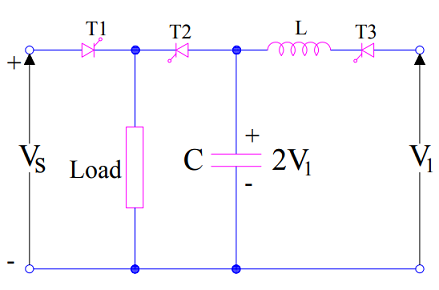# Class-E Commutation or External Pulse Commutation

Class-E Commutation is one of the forced commutation method to turn off an SCR / Thyristor. An external current pulse is used in this technique to commutate SCR. This is the reason, Class-E commutation is also known as External Pulse Commutation. This external current pulse is obtained form a separate voltage source. .

## Condition for Successful Commutation by Class-E Commutation Method:

The most important condition for reliable commutation of SCR by this technique is that, the magnitude of current pulse must be more than the load current.

## Class-E Commutation Technique:

Figure below shows the circuit diagram for External Pulse Commutation.In the figure,

• Vs is the main voltage source,
• T1 is main thyristor which carry the load current,
• V1 is the external voltage source to obtain
• T2 and T3 are auxiliary thyristors used for commutation of main thyristor T1
• L and C are Inductor and Capacitor respectively.

### Working Principle

Initially thyristor T1 is conducting and hence, load is being fed by main voltage source Vs through T1. Now, we want to turn off main SCR T1.

For this, thyristor T3 is fired or gated at any instant of time to turn it ON. Let us assume, this time to be t=0 sec. Once T3 is ON, it starts conducting at t=0 sec. A resonating circuit consisting of V1, L and C is formed and resonating current starts flowing. Due to resonating current, capacitor C gets charged up to 2V1 at t = π√(LC) with its upper plate positive as shown in figure. After t= π√(LC), capacitor will not allow any flow of resonating current as it is fully charged. Thus, the current through T3 gets reduced to zero and hence commutated.

Now, thyristor T2 is fired or gated to turn it ON. Once T2 is ON, it starts conducting. With T2 ON, main thyristor T1 is subjected to a reverse voltage. The magnitude of his reverse voltage is (Vs – 2V1). Therefore, main thyristor T1 is turned OFF.

After T1 is turned off or commutated, capacitor dissipated its stored energy through the load.

### 3 thoughts on “Class-E Commutation or External Pulse Commutation”

1.owen ezeagwula says:

Interesting topic.
Like to see more of commutation methods for SCR used in A.C networks and also the triggering devices and associated circuits.

1.admin says:

Thank you very much! Please share if you like it.

2.umesh sharma says: